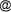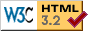## Evaluating Equations to Predict Organic Matter Digestibility Of tropical Ruminant Diets from Fecal Crude Protein Concentrations

### Anil Kumar Gajaga Krishnappa, Joaquín Castro-Montoya, Uta Dickhoefer

University of Hohenheim, Animal Nutrition and Pasture Management in the Tropics and Subtropics (490i), Germany

### Abstract

The apparent total tract organic matter digestibility (OMD) is one of the primary parameters to evaluate nutritive value of feed consumed by ruminants. Estimating OMD in vivo requires abundant work and the need to collect the totality of faeces voided by the animal. To facilitate this estimation, equations based on the crude protein concentration in faeces have been developed: 1) Wang equation = 0.899−0.644 × exp (−0.5774×faecal CP(g/kgOM)/100)), 2) Peripolli equation = 0.7326 −0.3598exp[(−0.9052 CP (g/kg OM))/100 and 3) Lukas equation =79.76 − 107.7e(−0.01515*cp) have been developed to predict OMD in domestic ruminants fed forage-based diets.Since the equations were developed under specific experimental conditions, the potential of these equations to accurately predict OMD under other settings needs to be verified.Therefore, the potential of all the three equations was evaluated by comparing OMD estimated by these equations with the in vivo OMD measured in diets using tropical forages obtained from literature. In total, 224 in vivo measured OMD from different experiments conducted in the Tropics with cattle, sheep, and goats were included. Diets were selected so that the forage portion contained either 100 % legume, 50 % legume+50 % grass, or 100 %grass. Calculated OMD were regressed on the measured OMD and the slope, intercept, and R square were estimated to evaluate the performance of the equations using R-studio statistical software. A total of 12 comparisons were conducted, all R square were below 0.23 and slopes and intercepts ranged from 0.21 to 0.58 and from 200.7 to 546.7, respectively. Hence, the ability of all three equations to predict accurately OMD is very limited regardless of the characteristics of the forage in the diet. These results indicate that the equations developed to predict OMD from faecal N cannot be applied with confidence in a variety of tropical feeding conditions.

Keywords: Digestibility, ruminants ,tropical feedstuffs

Contact Address: Anil Kumar Gajaga Krishnappa, University of Hohenheim, Animal Nutrition and Pasture Management in the Tropics and Subtropics (490i), Egilostrasse 45 0213, 70599 Stuttgart, Germany, e-mail: anilgk5009gmail.com